Next: Basic Concepts and Terminology Up: Glow Discharges in Inert Previous: Vacuum Technology   Contents   Index

## Electron and Ion Mobility

The concept of mobility is used in plasma physics, to describe the drift velocity of charged particles under the impact of an electric field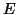. The significantly different mobility of electrons and ions in weakly ionized plasmas is of crucial importance for the understanding of fundamental issues like plasma potential and self bias voltage in materials processing. Due to this remarkable importance we will go a little deeper in that issue already here.

Let us assume a cloud of electrons subjected to an external electric field, which will accelerate them in opposite field direction. The electrons cannot continously aquire higher velocity because the collision probability with other particles is also increasing at higher speed. A steady state with some net drift velocitywill be established.

This is clear by recalling the following assumptions and facts, that we already partially considered.

• The discharges of our interest are weakly ionized, means the dominant collision partners for charged particles are neutrals, collisions between charged particles must not be considered.

There is an equilibrium where the average drift velocity is proportional to the applied field electric field: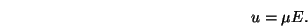(1.9)

The proportional constant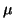is called mobility.

Nearly the complete power is transfered to the electrons due to the significant mass difference,denotes the electron and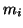the ion mass respectively. For illustration we will follow a simple argument given by Chapmann . Consider the example of a constant electric fieldacting on an initially stationary single charged particle. After a given time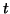the particle traveled the distance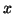, where the accelerationdepends on the magnitude of the field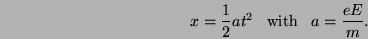Therefore the work done on the particle is given by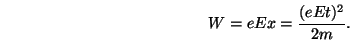As we can see the mass turns up in the denominator, resulting in a much higher energy transfer from the electric field to the light electrons compared to the ions, as stated above.Next: Basic Concepts and Terminology Up: Glow Discharges in Inert Previous: Vacuum Technology   Contents   Index

back to www.gs68.de

Other important things: Legal Notice, Contact us

Copyright © 2001-2012 Spitzlsperger Technologies GmbH